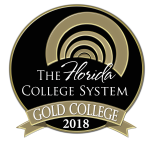MAT1033

## Intermediate Algebra

Course Code:
MAT1033

Credit Hours:
3 (elective credit)

Effective beginning:
2023-24

Sections:
001-003, 100

Course Description:
Topics in this course include: linear and quadratic equations, linear inequalities, systems of linear equations and inequalities in two variables, exponents, factoring, rational expressions and equations, radicals, complex numbers, introduction to functions, and applications.

### Course Details

##### Prerequisites:

A score of 114 – 122 on the PERT placement exam,

OR an acceptable score on ACT or SAT with successful completion of the equivalent of one year of Algebra I,

OR a “C” or higher in the high school course Math for College Success within the past two years, or consent of the department.

This is not a Gordon Rule course and does not satisfy part of the general education requirements in mathematics. A “C” grade or higher must be made in this course to advance to a higher-level mathematics course.

##### Instructors:

Donna Huff
huffd@chipola.edu

##### Required textbooks/ course materials:

Intermediate Algebra (Ohm Bundle) by Lumen Learning et al. (OER). ISBN 9781640870246

##### Assignment/course outline:

See your Instructor First Day Handout.

##### Discipline-level learning outcomes:

Area 3 -Math:   Demonstrate Basic Mathematical Skills and Knowledge

The purpose of the mathematics component of the core curriculum is to develop quantitative literacy with an ability to apply basic mathematical tools in the solution of real-world problems.

M-1 Solve mathematical problems by using arithmetic, algebraic, or geometric skills.

M-2 Translate basic mathematical information verbally, numerically, graphically, or symbolically.

M-3 Solve mathematical problems using appropriate technology.

M-4 Interpret mathematical models such as formulas, graphs, tables, or schematics.

M-5 Solve contextual problems using mathematical processes.

MAT 1033 is not a General Education core course and therefore a student in MAT 1033 will demonstrate selected learning outcomes from this list.

##### Course-level learning outcomes:

Course-level student learning outcomesDiscipline-level learning outcomesAssessment methods
• Apply properties of algebra to simplify, add, subtract, multiply and/or divide algebraic terms
• Calculate the factorization of various types of polynomials
• Solve various types of equations and inequalities
• Calculate the equation of a linear function
• Identify various characteristics related to the graph of a function such as domain or range
• Solve systems of equations and inequalities
• Solve problems in other disciplines by using mathematical processes

M-1, M-2, M-4, M-5

M-1, M-2, M-4

M-1, M-2, M-4, M-5

M-1, M-2, M-4, M-5

M-1, M-2, M-4, M-5

M-1, M-2, M-4, M-5

M-1, M-2, M-4, M-5

Homework, Unit Tests, Cumulative Final

##### Means of accomplishing learning outcomes:

• Teacher facilitated: The teacher will be leading class discussions on the material contained in the text during each class period.
• Student-centered: The students will take notes and practice solving problems during each class period.
• Office Hours: The instructor will be available during office hours for individual assistance. The instructor’s schedule can be found posted on their office door and/or via their individual web site.
• ACE tutors: Student tutors are available in the ACE to provide individualized help. Hours can be found posted each semester on the ACE door and/or via the web site.

### College-wide policies and resources

For more specific information on Chipola's college-wide academic policies and resources available to students, visit the link below.Chipola College
3094 Indian Circle
Marianna, FL 32446
850.526.2761